Next: Region Labeling Up: PREPROCESSING OF N-DIMENSIONAL DATA Previous: PREPROCESSING OF N-DIMENSIONAL DATA

Convolution

Both the smoothing and boundary detection procedures are instances of the convolution operation. The discrete case of 2-D convolution is done by taking two data matrices, one the original data set and the other a mask'', and effectively sliding'' one across the other, matching all the points of one against all the points of the other. The result is a new data matrix that has a maximum range in any given dimension that is the range of the original data set for that dimension plus the range of the mask, minus one. For example, if a data set with the range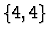were convolved with a mask with a range of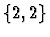, the new resulting data space would have a range of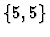. The convolution process multiplies corresponding elements between the mask and the original matrix and sums all of the products. Different configurations of masks produce different types of results.

This concept is easily extendible from two-dimensions into N-dimensions. The algorithm is exactly the same, extending over all the dimensions in the space. For example in four dimensions a data set of cardinality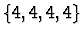convolved with a mask of cardinality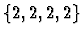produces a new data set with a range of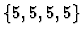. From this, the operations of smoothing and boundary detection can be implemented. Figures 6a and 6b show the effects of a using directional Sobel operator to detect boundaries on a 4-D hypersurface. The first figure shows that, while the boundaries have been detected, they do not appear to be cohesive. Manipulating the data as discussed in section 3.2 shows that what appear to be uniformly scattered points actually do form a connected boundary.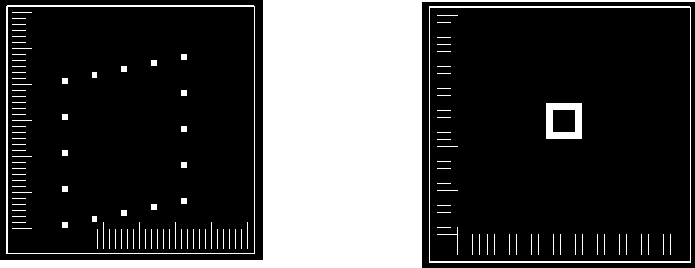Next: Region Labeling Up: PREPROCESSING OF N-DIMENSIONAL DATA Previous: PREPROCESSING OF N-DIMENSIONAL DATA
Matthew Ward
1999-02-23